StatLect

Indicator functions

The indicator function of an event is a random variable that takes value 1 when the event happens and value 0 when the event does not happen. Indicator functions are often used in probability theory to simplify notation and to prove theorems.Definition

The following is a formal definition.

Definition Letbe a sample space andbe an event. The indicator function (or indicator random variable) of the event, denoted by, is a random variable defined as follows: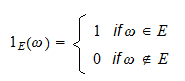While the indicator of an eventis usually denoted by, sometimes it is also denoted bywhereis the Greek letter Chi.

Example We toss a die and one of the six numbers from 1 to 6 can appear face up. The sample space isDefine the eventdescribed by the sentence "An even number appears face up". A random variable that takes value 1 when an even number appears face up and value 0 otherwise is an indicator of the event. The case-by-case definition of this indicator isFrom the above definition, it can easily be seen thatis a discrete random variable with supportand probability mass function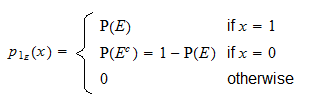Properties

Indicator functions enjoy the following properties.

Powers

The-th power ofis equal to:becausecan be eitherorandExpected value

The expected value ofis equal to: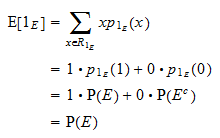Variance

The variance ofis equal to. Thanks to the usual variance formula and the powers property above, we obtain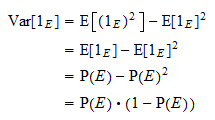Intersections

Ifandare two events, thenbecause:

1. if, thenand2. if, thenand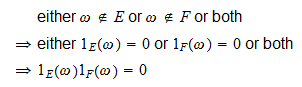Indicators of zero-probability events

Letbe a zero-probability event andan integrable random variable. Then,While a rigorous proof of this fact is beyond the scope of this introductory exposition, this property should be intuitive. The random variableis equal to zero for all sample pointsexcept possibly for the points. The expected value is a weighted average of the valuescan take on, where each value is weighted by its respective probability. The non-zero valuescan take on are weighted by zero probabilities, somust be zero.

Solved exercises

Below you can find some exercises with explained solutions.

Exercise 1

Consider a random variableand another random variabledefined as a function of.Expressusing the indicator functions of the eventsand.

Solution

Denote bythe indicator of the eventand denote bythe indicator of the event. We can writeas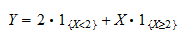Exercise 2

Letbe a positive random variable, that is, a random variable that can take on only positive values. Letbe a constant. Prove thatwhereis the indicator of the event.

Solution

First note that the sum of the indicatorsandis always equal to:As a consequence, we can write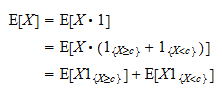Now, note thatis a positive random variable and that the expected value of a positive random variable is positive:Thus,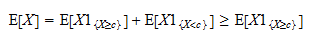Exercise 3

Letbe an event and denote its indicator function by. Letbe the complement ofand denote its indicator function by. Can you expressas a function of?

Solution

The sum of the two indicators is always equal to:Therefore,The book

Most of the learning materials found on this website are now available in a traditional textbook format.

Glossary entries
Share